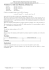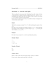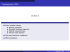# SFWR ENG 2DM3 Discrete Mathematics and Logic

## Transcription

SFWR ENG 2DM3 Discrete Mathematics and Logic
```SFWR ENG 2DM3
Discrete Mathematics and Logic
Solution Set 2
8 Questions
1. Prove that there are no integer solutions (x, y) to the equation 4x = y 2 + 1.
Solution:
First, we observe that any integer perfect square is equivalent to 0 or 1 modulo 4. To see why this is
true, we note that every integer is either odd or even, and can be expressed as 2k or 2k + 1 for some
integer k. So every perfect square can be expressed as (2k)2 = 4k 2 or (2k + 1)2 = 4k 2 + 4k + 1, which
are equivalent to 0 and 1 modulo 4, respectively.
Now, the left side of the given equation is clearly equivalent to 0 modulo 4 (since it is an integer
multiple of 4). But since y 2 is 0 or 1 modulo 4, y 2 + 1 can only possibly be 1 or 2 modulo 4. Therefore,
the left side and right side of the equation cannot be equivalent modulo 4; so no integer solutions (x, y)
can exist.
2. (a) What is the value of gcd(24, 36)?
(b) What is the value of lcm(24, 36)?
(c) (Page 273, Exercise 30). If the product of two integers is 27 38 52 711 and their greatest common
divisor is 23 34 5, what is their least common multiple?
Solution: For parts (a) and (b), it may be helpful to consider the prime factorization 24 and 36.
24 = 23 · 3
36 = 22 · 32
(a) The greatest common divisor of 24 and 36 consists of the prime factors common to both integers.
From each prime in the above prime factorization, we take the smallest exponent. gcd(24, 36) =
22 · 3 = 12.
(b) The least common multiple of 24 and 36 is the smallest integer that is a multiple of both 24 and
36. From each prime in the above prime factorization, we take the largest exponent. lcm(24, 36) =
23 · 32 = 72.
(c) Let the integers be a and b. An important property is that a · b = gcd(a, b) · lcm(a, b). This gives
lcm(a, b) =
27 38 52 711
a·b
=
= 24 34 5711 .
gcd(a, b)
23 34 5
Remark: We can see that this property is true in this case by considering the prime factorizations
of a and b:
a = 2a1 · 3a2 · 5a3 · 7a4
b = 2b1 · 3b2 · 5b3 · 7b4
The greatest common divisor can be found by taking the min of each exponent, the least common
multiple can be found by taking the max of each exponent, and the product can be found by
taking the sum of each exponent. (See page 266 of the text for reference). Given the product
a · b, dividing by gcd(a, b) gives each prime factor to the exponent of max(ai , bi ), which is the
least common multiple.
1
3. (Page 272, Exercise 12). Prove that for every positive integer n, there are n consecutive composite
integers. [Hint: Consider the n consecutive positive integers starting with (n + 1)! + 2.]
Solution: Given any positive integer n, we prove that each of the n consecutive integers
[(n + 1)! + 2], [(n + 1)! + 3], . . . , [(n + 1)! + (n + 1)]
is composite.
Each of these integers can be expressed as ai = (n + 1)! + i for i = 2, 3, . . . , (n + 1). Since (n + 1)! is a
product of integers less than or equal to (n + 1), (n + 1)! must be divisible by i. Since both (n + 1)!
and i are divisible by i, their sum must be divisible by i. Therefore, for all values i = 2, 3, . . . , (n + 1),
the value ai is divisible by i and is therefore composite.
4. (Page 379, Exercise 6). Use mathematical induction to show that
2n > n2 + n
whenever n is an integer greater than 4.
Solution:
Basis step:
For n = k, we have
25 > 52 + 5
⇔ 32 > 30
which is clearly true.
Inductive step:
As our induction hypothesis, we assume that 2k > k 2 + k. We wish to show that this implies 2k+1 >
(k + 1)2 + (k + 1).
2k > k 2 + k
⇒ 2k+1 > 2k 2 + 2k
Since (k − 2)(k + 1) ≥ 0, we have that k 2 − k − 2 ≥ 0 =⇒ k 2 ≥ k + 2. We can substitute this into our
inequality:
2k+1 > 2k 2 + 2k
= k 2 + k 2 + 2k
≥ k 2 + (k + 2) + 2k
= k 2 + 3k + 2
= k 2 + 2k + 1 + k + 1
= (k + 1)2 + (k + 1)
This gives us 2k+1 > (k + 1)2 + (k + 1), as required. This completes our induction.
5. (a) List all of the ways to rearrange the characters in the word CAT.
(b) How many ways are there to rearrange the letters in the word MISSISSAUGA?
Solution:
(a) CAT, CTA, ACT, ATC, TAC, TCA
2
(b) There are 11 characters with two I’s, 4 S’s, and 2 A’s. This means the number of ways is
11!
= 415800
2!4!2!
6. (a) In how many ways can ten members of a wedding party stand in a line?
(b) In how many ways can ten members of a wedding stand in a line, such that the bride and groom
are standing next to each other?
Solution:
(a) This is equivalent to finding the number of ways to rearrange ten distinct characters in a string,
equal to 10! = 3628800.
(b) We can group together the bride and groom as if they were one person, count the number of ways
to arrange the 9 ‘people’, then multiply 2! to this number since their order can be switched with
each other. This gives 9! × 2 = 725760.
7. (Page 397, Exercise 44). Given a group of ten distinct people, how many ways are there to seat four
of them around a circular table? (Two seatings are considered the same when everyone has the same
immediate left and immediate right neighbour).
Solution:
We need to count the number of ways to select four people out of ten, then the number of ways to
rearrange them in a circle.
The number of ways to select four people from ten is 10
4 = 210.
Since the order around the circle is insensitive to rotation, we can count the number of arrangements
of four people around a circle by fixing one and counting the ways to order the remaining three. Each
such arrangement can be represented as a string with three unique letters, with the nth position of
the string representing the identity of the person sitting n spots to the fixed person’s right. This gives
3! = 6 arrangements.
So the number of ways to seat four people out of ten in a circle is
10
× 3! = 1260.
4
8. (Page 422, Exercise 28). Show, through either a combinatorial argument or algebraic manipulation,
that if n is a positive integer:
2n
n
=2
+ n2
2
2
Solution:
Combinatorial Argument:
Suppose there are 2n people in a room, with n girls and n boys. The left side counts the number of
ways to select 2 people from the 2n people in the room. The right side can be written as
n
n
+
+n×n
2
2
which is a sum of the number of ways to select two boys (equal to n2 ), the number of ways to select
two girls (equal to n2 ), and the number of ways to select one boy and one girl (equal to n × n). So the
left side and right side of this equation correspond to the same counting problem, and will therefore
have the same value.
3
Algebraic Manipulation:
Recall that
n
n!
n(n − 1)
=
=
2
(n − 2)!2!
2
So the left side equals
2n(2n−1)
2
= 2n2 − n. The right side is
n
2n(n − 1)
2
+ n2 =
+ n2
2
2
= n2 − n + n2
= 2n2 − n
which equals the left side.
4
```

### Homework 3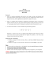### Discrete Math, Spring 2013 - Some Sample Problems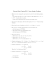### 2013 School Competition Countdown Round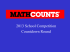### Sample Individual Questions 2012 Canadian Team Mathematics Contest (CTMC)### Elementary Number Theory and Cryptography, Michaelmas 2014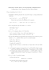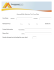### Even and Odd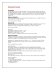### SAMPLE PAPER-2014 CLASS-X Subject : Maths TIME: 3 hours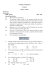### CSCE 411 Sample Midterm Exam 1. (10 points for each part)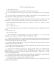### Homework solutions - California State University, Los Angeles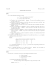### Sample Problems Algebra### CENG 277 Discrete Structures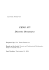### X Copa UCI de ProgramaciÃ³n "TomÃ¡s LÃ³pez JimÃ©nez"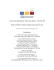### Div2 Problems (Eng)